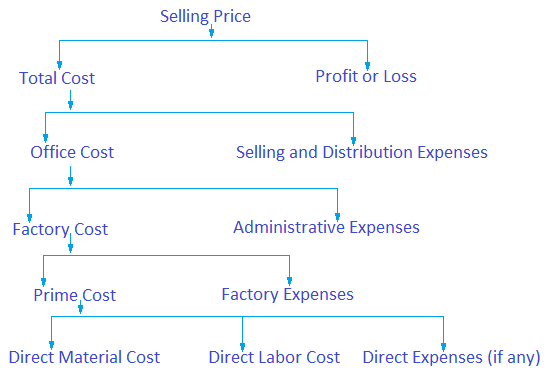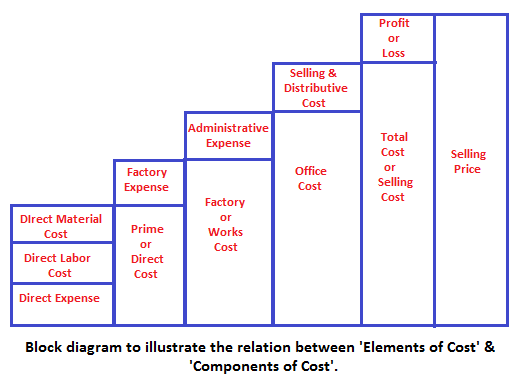# Component Cost

The various components of cost are:

1. Prime cost
2. Factory cost
3. Office cost
4. Total cost

## Prime Cost

It consists of direct material cost, direct labor cost and direct expenses.

i.e. Prime cost = Direct material cost + Direct labor cost + Direct expenses.

Prime cost is also named as Direct Cost.

## Factory Cost

It consists of prime cost and factory expenses.

i.e. Factory cost = Prime cost + Factory expenses.

Factory cost is also named as works cost.

## Office Cost

It consists of factory cost and administrative expenses.

i.e Office cost = Factory cost + Administrative expenses.

Office cost is also named as manufacturing cost or cost of production.

## Total Cost

It includes office cost, and selling and distribution expenses.

i.e. Total Cost = Office cost + Selling expenses + Distribution expenses.

## Selling Price

If the profit is added in the total cost of the product it is called selling price. The customers get the products by paying the price which is named as selling price.

The relation between the elements of cost and components of cost can be best illustrated by the chart given below.

The relation between the elements of cost and components of cost can be best illustrated by the chart given below.This can also be illustrated by the block diagram.Next Chapters

Latest Articles

Average Acceleration Calculator

Average acceleration is the object's change in speed for a specific given time period. ...

Free Fall Calculator

When an object falls into the ground due to planet's own gravitational force is known a...

Permutation

In Mathematics, the permutation can be explained as the arrangement of objects in a particular order. It is an ordered...

Perimeter of Rectangle

A rectangle can be explained as a 4-sided quadrilateral which contains equal opposite sides. In a rectangle

Perimeter of Triangle

A three sided polygon which has three vertices and three angles is called a triangle. Equilateral triangle...